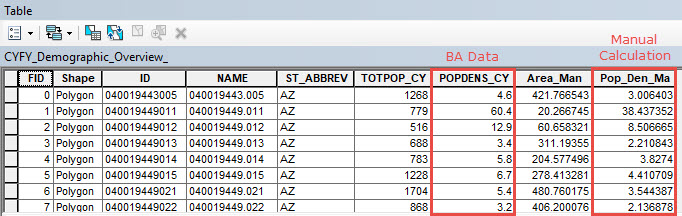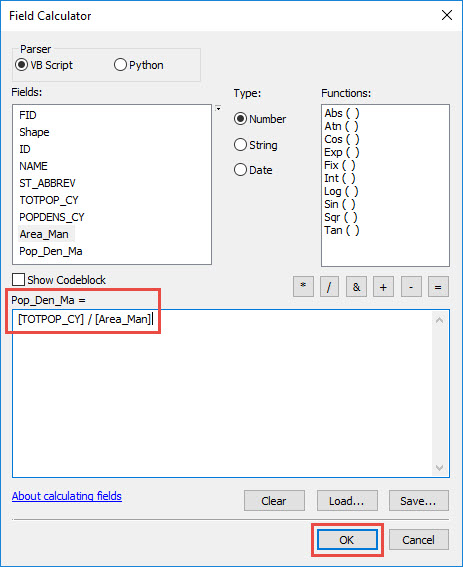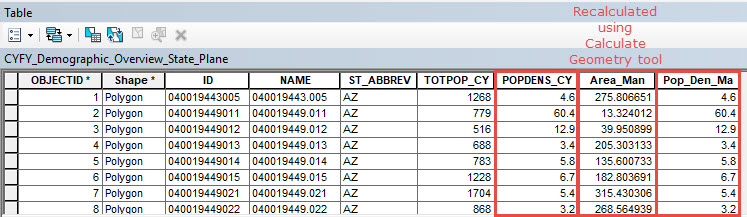English

# Problem: Discrepancy in Business Analyst population density data and the population density calculated manually

## Description

Business Analyst Desktop may be used to obtain the population density of an area. Population density data are required to determine the growth of a population, for example.

However, the population density calculated manually using the Field Calculator returns a different value compared to the population density data recorded in Business Analyst Desktop.## Cause

The discrepancy in the values is due to the different coordinate system used to calculate the data. The Business Analyst Desktop population density data is recorded using the State Plane Coordinate System. In the manual calculation, the area populated in the attribute table is calculated using the Calculate Geometry tool. The tool's data frame setting uses the WGS 1984 Web Mercator coordinate system by default.

## Solution or Workaround

To obtain identical population density values, follow the instructions described below:

1. Use the Project tool to project the Business Analyst Desktop dataset to a State Plane Coordinate System.
2. Open the attribute table of the new projected layer.
3. Right-click the area field and click Calculate Geometry.
4. In the Calculate Geometry dialog box, set the Property to Area and set the Units to Square Miles US [sq mi]. Click OK.
5. Calculate the Population Density:
1. Right-click the existing area field and click Field Calculator.
2. In the Field Calculator dialog box, divide the population field by the area calculated using the Calculate Geometry, above, and click OK.